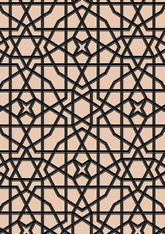data198/AL2

## Geometry

• The symmetry group of the tiling is *442 (p4m).
• All the internal angles of the constituent polygons are a multiple of 30°.
• Contains five equilateral triangles.
• Contains one regular hexagon.
• Contains two regular four-pointed star polygons with vertex angle of 30°.
• There are 6 non-regular reflective tiles (including 2 kites).
• The tiling satisfies the two-colour condition.
• The tiling is edge-to-edge.
• As drawn, contains about 473 polygons.

## References

Publications referenced: